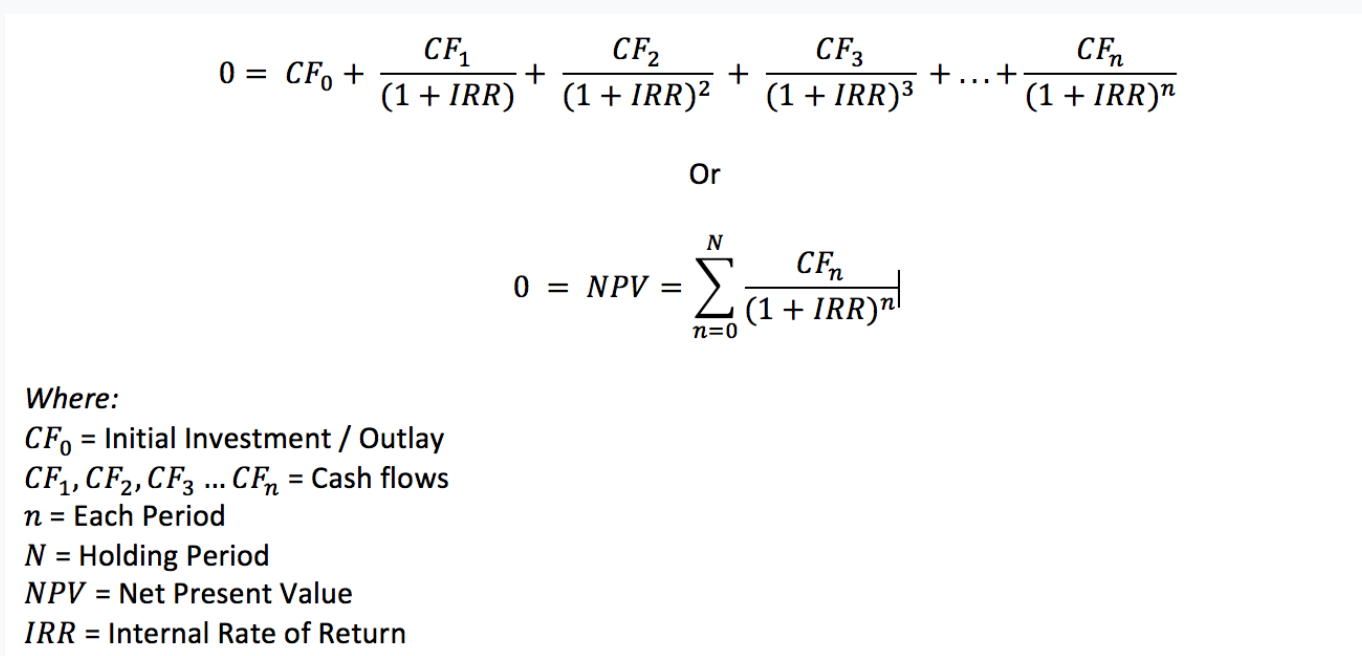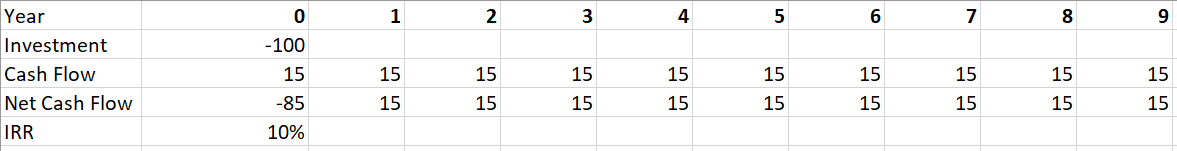# How to properly compute the ROI of a project with several cashflows?

opportunity assessment ROI calculation
New answer on May 01, 2020
1.2 k Views

Hello everyone,

I recently came accross a case with an ROI computation (with multi-year cashflows) and was wondering what would be the proper/best method for it, especially how to factor in the time value of money.

Indeed, here, we had an initial investment of 100MUSD and a cashflow of 15MUSD every year during 10 years (starting year 0).

What would be the best method for computing the ROI :

(i) simply sum up all 10 years cashflows WITHOUT considering time value of money (10 x 15 MUSD - 100MUSD of initial investment), divided by investment (100 MUSD) => 50% ROI ;

(ii) or factoring in the time value of money, by "discounting" the several Cashflows , deriving an NPV and dividing this NPV by initial investment. Here, it means we have to determine the right discount rate for discounting.

Indeed, I would rather go for first approach, but that seems quite "incomplete" since we have multiple years cashflows, and time is not taken into account.

The next step in the case is then to compare several projects and pick the best one. Here, we have 2 projects with same ROI (based on method (i)). However, the timespan of the cashflows are not the same (first project -> cashflows spread over a period of 5 years, second project -> cashflows spread over a period of 10 years). I this case, I would rather go for the 1st project (the sooner the cashflow, the better).

Thank you.

Best,

Clara

## Overview of answers

• Date ascending
• Date descending

Hi Clara,

Great question, thanks for reaching out!

First, let's agree what we mean by ROI and why we want to calculate it. In this simplest case ROI is the same as IRR (because it’s a project with a fixed timeline), it's an annualized rate of return that we're going to get on our investment. We usually want to calculate it to compare with our Cost of Capital or “WACC” (which is the time value of money for the investor). In conclusion, if our return exceeds our cost of capital, that means we're creating value for our shareholders. So you don’t use time value of money when calculation ROI, you rather use it as a comparable value.

Using the above logic, if the return exactly equals cost of capital we’re not creating and not destroying value, so the NPV is exactly 0. And that’s the equation you use to calculate the IRR (you discount your cashflows by exactly IRR and solve for NPV=0):For the case interviews no one expects you to be able to nail an IRR number in your head. But if you can get pretty close, you're fine. There are some simple IRRs that you can remember, such as:

• Double your money in 1 year, IRR = 100%
• Double your money in 2 years, IRR = 41%; about 40%
• Double your money in 3 years, IRR = 26%; about 25%
• Double your money in 4 years, IRR = 19%; about 20%
• Double your money in 5 years, IRR = 15%; about 15%

For more than 5 years, there's also a "Rule of 72", which says that if you divide 72 by your rate of return, the resulting number is roughly how many years it will take your money to double. For example, if I expect returns of 7 percent a year, I would expect my money to double in about 10 years (72 / 7 = 10.3 years).

Let's look at your case. We invest 100 and get 15 every year, so it takes around 7 years to "double our money" (in this case of a fixed project - to return our 100 back). By the rule of 72, we can say that the IRR is 72/7 ~= 10%, which is pretty close if you want to double-check it in Excel:Now, on the second part you are absolutely right. If the projects have the same IRR and require the same investment, you want your cashflows as soon as possible, so you are comparing your Payback periods (how long it takes to return the initial investment).

Hope this helps, feel free to reach out if you have any further questions.

Best regards,

Vasily

(edited)

Hi Clara,

I guess what you are referring in your questions is the NPV and not the ROI. Answering your questions:

1. You should clarify that with the interviewer. For simplicity, most of the time no discount rate is fine, but you should make clear to the interviewer you thought about it
2. To assess the best project you should also consider
1. Possible synergies
2. Capabilities required to start the projects

If they are the same, then it would make sense to go with option 1

Best,

Francesco